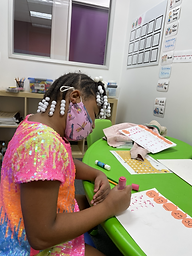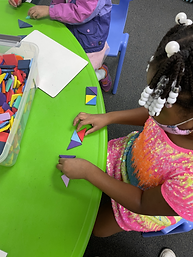## Ms. Caitlyn

### Target 1​

###### Lesson Type:

New

Algebra

:

Sequence

Use arithmetic to define a sequence and calculate the next unit in a series.

###### 1:

Study a given arithmetic sequence to determine what rule explains the sequence (i.e., 2-4-6-8-10…each number is the sequence gets bigger by 2).

###### 2:

Write an arithmetic rule to explain a given sequence.

###### 3:

Understand that a pattern can be described by stating the rule that it is following.

1st

###### Vocabulary:

Sequence, Arithmetic Rule

Activities:

Students identified the rules of arithmetic sequences that they were given.

Students completed the missing number in sequences.

Students made a "pumpkin patch" and wrote how much each sequence grew on the vines.### Home Exploration

###### Guiding Questions:## Absent Students:

Ayden

### Target 2

:

###### 1:

Identify and complete a pattern of rotation.

###### 2:

Identify and complete a growing pattern, where something new is added to each segment.

1st

###### Vocabulary:

Growing Pattern, Rotational Pattern

Activities:

Students filled in the missing parts of patterns given to them by the teacher.

Students filled in the missing parts of each other's patterns.### Home Exploration

###### Guiding Questions:### Target 3

:

###### 1:

Construct patterns of rotation.

###### 2:

Construct growing patterns.

1st

###### Vocabulary:

Growing Pattern, Rotational Pattern

Activities:

Students made growing patterns using foam shapes.

Students made rotational patterns using foam shapes.

Students traced stencils to form rotational patterns.### Home Exploration